Next: Electrostatics Up: Time-dependent Maxwell's equations Previous: Retarded fields

## Summary

This marks the end of our theoretical investigation of Maxwell's equations. Let us now summarize what we have learned so far. The field equations which govern electric and magnetic fields are written: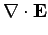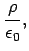(556)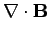(557)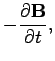(558)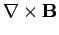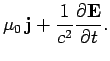(559)

These equations can be integrated to give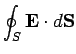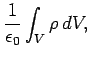(560)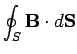(561)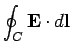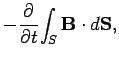(562)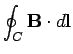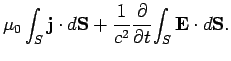(563)

Equations (557) and (558) are automatically satisfied by writing(564)(565)

This prescription is not unique (there are many choices of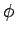andwhich generate the same fields) but we can make it unique by adopting the following conventions:(566)

and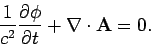(567)

Equations (556) and (559) reduce to(568)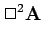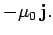(569)

These are driven wave equations of the general form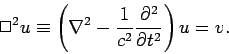(570)

The Green's function for this equation which satisfies the boundary conditions and is consistent with causality is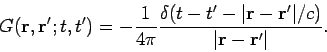(571)

Thus, the solutions to Eqs. (568) and (569) are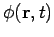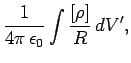(572)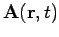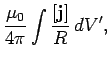(573)

where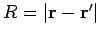, and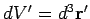, with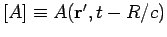. These solutions can be combined with Eqs. (564) and (565) to give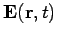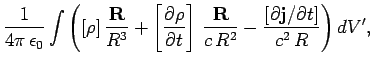(574)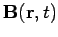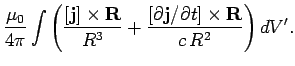(575)

Equations (556)-(575) constitute the complete theory of classical electromagnetism. We can express the same information in terms of field equations [Eqs. (556)-(559)], integrated field equations [Eqs. (560)-(563)], retarded electromagnetic potentials [Eqs. (572) and (573)], and retarded electromagnetic fields [Eqs. (574) and (575)]. Let us now consider the applications of this theory.Next: Electrostatics Up: Time-dependent Maxwell's equations Previous: Retarded fields
Richard Fitzpatrick 2006-02-02